## 1.3  Formulating Abstractions with Higher-Order Procedures

We have seen that procedures are, in effect, abstractions that describe compound operations on numbers independent of the particular numbers. For example, when we

(define (cube x) (* x x x))

we are not talking about the cube of a particular number, but rather about a method for obtaining the cube of any number. Of course we could get along without ever defining this procedure, by always writing expressions such as

(* 3 3 3)
(* x x x)
(* y y y)

and never mentioning cube explicitly. This would place us at a serious disadvantage, forcing us to work always at the level of the particular operations that happen to be primitives in the language (multiplication, in this case) rather than in terms of higher-level operations. Our programs would be able to compute cubes, but our language would lack the ability to express the concept of cubing. One of the things we should demand from a powerful programming language is the ability to build abstractions by assigning names to common patterns and then to work in terms of the abstractions directly. Procedures provide this ability. This is why all but the most primitive programming languages include mechanisms for defining procedures.

Yet even in numerical processing we will be severely limited in our ability to create abstractions if we are restricted to procedures whose parameters must be numbers. Often the same programming pattern will be used with a number of different procedures. To express such patterns as concepts, we will need to construct procedures that can accept procedures as arguments or return procedures as values. Procedures that manipulate procedures are called higher-order procedures. This section shows how higher-order procedures can serve as powerful abstraction mechanisms, vastly increasing the expressive power of our language.

### 1.3.1  Procedures as Arguments

Consider the following three procedures. The first computes the sum of the integers from a through b:

(define (sum-integers a b)
(if (> a b)
0
(+ a (sum-integers (+ a 1) b))))

The second computes the sum of the cubes of the integers in the given range:

(define (sum-cubes a b)
(if (> a b)
0
(+ (cube a) (sum-cubes (+ a 1) b))))

The third computes the sum of a sequence of terms in the series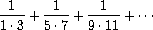which converges to/8 (very slowly):49

(define (pi-sum a b)
(if (> a b)
0
(+ (/ 1.0 (* a (+ a 2))) (pi-sum (+ a 4) b))))

These three procedures clearly share a common underlying pattern. They are for the most part identical, differing only in the name of the procedure, the function of a used to compute the term to be added, and the function that provides the next value of a. We could generate each of the procedures by filling in slots in the same template:

(define (<name> a b)
(if (> a b)
0
(+ (<term> a)
(<name> (<next> a) b))))

The presence of such a common pattern is strong evidence that there is a useful abstraction waiting to be brought to the surface. Indeed, mathematicians long ago identified the abstraction of summation of a series and invented ``sigma notation,'' for example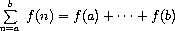to express this concept. The power of sigma notation is that it allows mathematicians to deal with the concept of summation itself rather than only with particular sums -- for example, to formulate general results about sums that are independent of the particular series being summed.

Similarly, as program designers, we would like our language to be powerful enough so that we can write a procedure that expresses the concept of summation itself rather than only procedures that compute particular sums. We can do so readily in our procedural language by taking the common template shown above and transforming the ``slots'' into formal parameters:

(define (sum term a next b)
(if (> a b)
0
(+ (term a)
(sum term (next a) next b))))

Notice that sum takes as its arguments the lower and upper bounds a and b together with the procedures term and next. We can use sum just as we would any procedure. For example, we can use it (along with a procedure inc that increments its argument by 1) to define sum-cubes:

(define (inc n) (+ n 1))
(define (sum-cubes a b)
(sum cube a inc b))

Using this, we can compute the sum of the cubes of the integers from 1 to 10:

(sum-cubes 1 10)
3025

With the aid of an identity procedure to compute the term, we can define sum-integers in terms of sum:

(define (identity x) x)

(define (sum-integers a b)
(sum identity a inc b))

Then we can add up the integers from 1 to 10:

(sum-integers 1 10)
55

We can also define pi-sum in the same way:50

(define (pi-sum a b)
(define (pi-term x)
(/ 1.0 (* x (+ x 2))))
(define (pi-next x)
(+ x 4))
(sum pi-term a pi-next b))

Using these procedures, we can compute an approximation to:

(* 8 (pi-sum 1 1000))
3.139592655589783

Once we have sum, we can use it as a building block in formulating further concepts. For instance, the definite integral of a function f between the limits a and b can be approximated numerically using the formula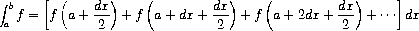for small values of dx. We can express this directly as a procedure:

(define (integral f a b dx)
(define (add-dx x) (+ x dx))
(* (sum f (+ a (/ dx 2.0)) add-dx b)
dx))
(integral cube 0 1 0.01)
.24998750000000042
(integral cube 0 1 0.001)
.249999875000001

(The exact value of the integral of cube between 0 and 1 is 1/4.)

Exercise 1.29.  Simpson's Rule is a more accurate method of numerical integration than the method illustrated above. Using Simpson's Rule, the integral of a function f between a and b is approximated as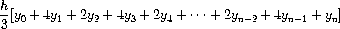where h = (b - a)/n, for some even integer n, and yk = f(a + kh). (Increasing n increases the accuracy of the approximation.) Define a procedure that takes as arguments f, a, b, and n and returns the value of the integral, computed using Simpson's Rule. Use your procedure to integrate cube between 0 and 1 (with n = 100 and n = 1000), and compare the results to those of the integral procedure shown above.

Exercise 1.30.  The sum procedure above generates a linear recursion. The procedure can be rewritten so that the sum is performed iteratively. Show how to do this by filling in the missing expressions in the following definition:

(define (sum term a next b)
(define (iter a result)
(if <??>
<??>
(iter <??> <??>)))
(iter <??> <??>))

Exercise 1.31.
a.  The sum procedure is only the simplest of a vast number of similar abstractions that can be captured as higher-order procedures.51 Write an analogous procedure called product that returns the product of the values of a function at points over a given range. Show how to define factorial in terms of product. Also use product to compute approximations tousing the formula52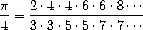b.  If your product procedure generates a recursive process, write one that generates an iterative process. If it generates an iterative process, write one that generates a recursive process.

Exercise 1.32.  a. Show that sum and product (exercise 1.31) are both special cases of a still more general notion called accumulate that combines a collection of terms, using some general accumulation function:

(accumulate combiner null-value term a next b)

Accumulate takes as arguments the same term and range specifications as sum and product, together with a combiner procedure (of two arguments) that specifies how the current term is to be combined with the accumulation of the preceding terms and a null-value that specifies what base value to use when the terms run out. Write accumulate and show how sum and product can both be defined as simple calls to accumulate.

b. If your accumulate procedure generates a recursive process, write one that generates an iterative process. If it generates an iterative process, write one that generates a recursive process.

Exercise 1.33.  You can obtain an even more general version of accumulate (exercise 1.32) by introducing the notion of a filter on the terms to be combined. That is, combine only those terms derived from values in the range that satisfy a specified condition. The resulting filtered-accumulate abstraction takes the same arguments as accumulate, together with an additional predicate of one argument that specifies the filter. Write filtered-accumulate as a procedure. Show how to express the following using filtered-accumulate:

a. the sum of the squares of the prime numbers in the interval a to b (assuming that you have a prime? predicate already written)

b. the product of all the positive integers less than n that are relatively prime to n (i.e., all positive integers i < n such that GCD(i,n) = 1).

### 1.3.2  Constructing Procedures Using Lambda

In using sum as in section 1.3.1, it seems terribly awkward to have to define trivial procedures such as pi-term and pi-next just so we can use them as arguments to our higher-order procedure. Rather than define pi-next and pi-term, it would be more convenient to have a way to directly specify ``the procedure that returns its input incremented by 4'' and ``the procedure that returns the reciprocal of its input times its input plus 2.'' We can do this by introducing the special form lambda, which creates procedures. Using lambda we can describe what we want as

(lambda (x) (+ x 4))

and

(lambda (x) (/ 1.0 (* x (+ x 2))))

Then our pi-sum procedure can be expressed without defining any auxiliary procedures as

(define (pi-sum a b)
(sum (lambda (x) (/ 1.0 (* x (+ x 2))))
a
(lambda (x) (+ x 4))
b))

Again using lambda, we can write the integral procedure without having to define the auxiliary procedure add-dx:

(define (integral f a b dx)
(* (sum f
(+ a (/ dx 2.0))
(lambda (x) (+ x dx))
b)
dx))

In general, lambda is used to create procedures in the same way as define, except that no name is specified for the procedure:

(lambda (<formal-parameters>) <body>)

The resulting procedure is just as much a procedure as one that is created using define. The only difference is that it has not been associated with any name in the environment. In fact,

(define (plus4 x) (+ x 4))

is equivalent to

(define plus4 (lambda (x) (+ x 4)))

We can read a lambda expression as follows:

(lambda             (x)             (+    x     4))the procedure   of an argument x  that adds  x and 4

Like any expression that has a procedure as its value, a lambda expression can be used as the operator in a combination such as

((lambda (x y z) (+ x y (square z))) 1 2 3)
12

or, more generally, in any context where we would normally use a procedure name.53

#### Using let to create local variables

Another use of lambda is in creating local variables. We often need local variables in our procedures other than those that have been bound as formal parameters. For example, suppose we wish to compute the function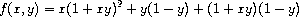which we could also express as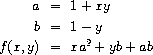In writing a procedure to compute f, we would like to include as local variables not only x and y but also the names of intermediate quantities like a and b. One way to accomplish this is to use an auxiliary procedure to bind the local variables:

(define (f x y)
(define (f-helper a b)
(+ (* x (square a))
(* y b)
(* a b)))
(f-helper (+ 1 (* x y))
(- 1 y)))

Of course, we could use a lambda expression to specify an anonymous procedure for binding our local variables. The body of f then becomes a single call to that procedure:

(define (f x y)
((lambda (a b)
(+ (* x (square a))
(* y b)
(* a b)))
(+ 1 (* x y))
(- 1 y)))

This construct is so useful that there is a special form called let to make its use more convenient. Using let, the f procedure could be written as

(define (f x y)
(let ((a (+ 1 (* x y)))
(b (- 1 y)))
(+ (* x (square a))
(* y b)
(* a b))))

The general form of a let expression is

(let ((<var1> <exp1>)
(<var2> <exp2>)(<varn> <expn>))
<body>)

which can be thought of as saying

 let have the value and have the value andhave the value in

The first part of the let expression is a list of name-expression pairs. When the let is evaluated, each name is associated with the value of the corresponding expression. The body of the let is evaluated with these names bound as local variables. The way this happens is that the let expression is interpreted as an alternate syntax for

((lambda (<var1...<varn>)
<body>)
<exp1><expn>)

No new mechanism is required in the interpreter in order to provide local variables. A let expression is simply syntactic sugar for the underlying lambda application.

We can see from this equivalence that the scope of a variable specified by a let expression is the body of the let. This implies that:

• Let allows one to bind variables as locally as possible to where they are to be used. For example, if the value of x is 5, the value of the expression

(+ (let ((x 3))
(+ x (* x 10)))
x)

is 38. Here, the x in the body of the let is 3, so the value of the let expression is 33. On the other hand, the x that is the second argument to the outermost + is still 5.

• The variables' values are computed outside the let. This matters when the expressions that provide the values for the local variables depend upon variables having the same names as the local variables themselves. For example, if the value of x is 2, the expression

(let ((x 3)
(y (+ x 2)))
(* x y))

will have the value 12 because, inside the body of the let, x will be 3 and y will be 4 (which is the outer x plus 2).

Sometimes we can use internal definitions to get the same effect as with let. For example, we could have defined the procedure f above as

(define (f x y)
(define a (+ 1 (* x y)))
(define b (- 1 y))
(+ (* x (square a))
(* y b)
(* a b)))

We prefer, however, to use let in situations like this and to use internal define only for internal procedures.54

Exercise 1.34.  Suppose we define the procedure

(define (f g)
(g 2))

Then we have

(f square)
4

(f (lambda (z) (* z (+ z 1))))
6

What happens if we (perversely) ask the interpreter to evaluate the combination (f f)? Explain.

### 1.3.3  Procedures as General Methods

We introduced compound procedures in section 1.1.4 as a mechanism for abstracting patterns of numerical operations so as to make them independent of the particular numbers involved. With higher-order procedures, such as the integral procedure of section 1.3.1, we began to see a more powerful kind of abstraction: procedures used to express general methods of computation, independent of the particular functions involved. In this section we discuss two more elaborate examples -- general methods for finding zeros and fixed points of functions -- and show how these methods can be expressed directly as procedures.

#### Finding roots of equations by the half-interval method

The half-interval method is a simple but powerful technique for finding roots of an equation f(x) = 0, where f is a continuous function. The idea is that, if we are given points a and b such that f(a) < 0 < f(b), then f must have at least one zero between a and b. To locate a zero, let x be the average of a and b and compute f(x). If f(x) > 0, then f must have a zero between a and x. If f(x) < 0, then f must have a zero between x and b. Continuing in this way, we can identify smaller and smaller intervals on which f must have a zero. When we reach a point where the interval is small enough, the process stops. Since the interval of uncertainty is reduced by half at each step of the process, the number of steps required grows as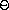(log( L/T)), where L is the length of the original interval and T is the error tolerance (that is, the size of the interval we will consider ``small enough''). Here is a procedure that implements this strategy:

(define (search f neg-point pos-point)
(let ((midpoint (average neg-point pos-point)))
(if (close-enough? neg-point pos-point)
midpoint
(let ((test-value (f midpoint)))
(cond ((positive? test-value)
(search f neg-point midpoint))
((negative? test-value)
(search f midpoint pos-point))
(else midpoint))))))

We assume that we are initially given the function f together with points at which its values are negative and positive. We first compute the midpoint of the two given points. Next we check to see if the given interval is small enough, and if so we simply return the midpoint as our answer. Otherwise, we compute as a test value the value of f at the midpoint. If the test value is positive, then we continue the process with a new interval running from the original negative point to the midpoint. If the test value is negative, we continue with the interval from the midpoint to the positive point. Finally, there is the possibility that the test value is 0, in which case the midpoint is itself the root we are searching for.

To test whether the endpoints are ``close enough'' we can use a procedure similar to the one used in section 1.1.7 for computing square roots:55

(define (close-enough? x y)
(< (abs (- x y)) 0.001))

Search is awkward to use directly, because we can accidentally give it points at which f's values do not have the required sign, in which case we get a wrong answer. Instead we will use search via the following procedure, which checks to see which of the endpoints has a negative function value and which has a positive value, and calls the search procedure accordingly. If the function has the same sign on the two given points, the half-interval method cannot be used, in which case the procedure signals an error.56

(define (half-interval-method f a b)
(let ((a-value (f a))
(b-value (f b)))
(cond ((and (negative? a-value) (positive? b-value))
(search f a b))
((and (negative? b-value) (positive? a-value))
(search f b a))
(else
(error "Values are not of opposite sign" a b)))))

The following example uses the half-interval method to approximateas the root between 2 and 4 of sin x = 0:

(half-interval-method sin 2.0 4.0)
3.14111328125

Here is another example, using the half-interval method to search for a root of the equation x3 - 2x - 3 = 0 between 1 and 2:

(half-interval-method (lambda (x) (- (* x x x) (* 2 x) 3))
1.0
2.0)
1.89306640625

#### Finding fixed points of functions

A number x is called a fixed point of a function f if x satisfies the equation f(x) = x. For some functions f we can locate a fixed point by beginning with an initial guess and applying f repeatedly,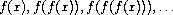until the value does not change very much. Using this idea, we can devise a procedure fixed-point that takes as inputs a function and an initial guess and produces an approximation to a fixed point of the function. We apply the function repeatedly until we find two successive values whose difference is less than some prescribed tolerance:

(define tolerance 0.00001)
(define (fixed-point f first-guess)
(define (close-enough? v1 v2)
(< (abs (- v1 v2)) tolerance))
(define (try guess)
(let ((next (f guess)))
(if (close-enough? guess next)
next
(try next))))
(try first-guess))

For example, we can use this method to approximate the fixed point of the cosine function, starting with 1 as an initial approximation:57

(fixed-point cos 1.0)
.7390822985224023

Similarly, we can find a solution to the equation y = sin y + cos y:

(fixed-point (lambda (y) (+ (sin y) (cos y)))
1.0)
1.2587315962971173

The fixed-point process is reminiscent of the process we used for finding square roots in section 1.1.7. Both are based on the idea of repeatedly improving a guess until the result satisfies some criterion. In fact, we can readily formulate the square-root computation as a fixed-point search. Computing the square root of some number x requires finding a y such that y2 = x. Putting this equation into the equivalent form y = x/y, we recognize that we are looking for a fixed point of the function58 yx/y, and we can therefore try to compute square roots as

(define (sqrt x)
(fixed-point (lambda (y) (/ x y))
1.0))

Unfortunately, this fixed-point search does not converge. Consider an initial guess y1. The next guess is y2 = x/y1 and the next guess is y3 = x/y2 = x/(x/y1) = y1. This results in an infinite loop in which the two guesses y1 and y2 repeat over and over, oscillating about the answer.

One way to control such oscillations is to prevent the guesses from changing so much. Since the answer is always between our guess y and x/y, we can make a new guess that is not as far from y as x/y by averaging y with x/y, so that the next guess after y is (1/2)(y + x/y) instead of x/y. The process of making such a sequence of guesses is simply the process of looking for a fixed point of y(1/2)(y + x/y):

(define (sqrt x)
(fixed-point (lambda (y) (average y (/ x y)))
1.0))

(Note that y = (1/2)(y + x/y) is a simple transformation of the equation y = x/y; to derive it, add y to both sides of the equation and divide by 2.)

With this modification, the square-root procedure works. In fact, if we unravel the definitions, we can see that the sequence of approximations to the square root generated here is precisely the same as the one generated by our original square-root procedure of section 1.1.7. This approach of averaging successive approximations to a solution, a technique we that we call average damping, often aids the convergence of fixed-point searches.

Exercise 1.35.  Show that the golden ratio(section 1.2.2) is a fixed point of the transformation x1 + 1/x, and use this fact to computeby means of the fixed-point procedure.

Exercise 1.36.  Modify fixed-point so that it prints the sequence of approximations it generates, using the newline and display primitives shown in exercise 1.22. Then find a solution to xx = 1000 by finding a fixed point of xlog(1000)/log(x). (Use Scheme's primitive log procedure, which computes natural logarithms.) Compare the number of steps this takes with and without average damping. (Note that you cannot start fixed-point with a guess of 1, as this would cause division by log(1) = 0.)

Exercise 1.37.  a. An infinite continued fraction is an expression of the form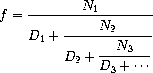As an example, one can show that the infinite continued fraction expansion with the Ni and the Di all equal to 1 produces 1/, whereis the golden ratio (described in section 1.2.2). One way to approximate an infinite continued fraction is to truncate the expansion after a given number of terms. Such a truncation -- a so-called k-term finite continued fraction -- has the form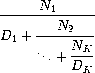Suppose that n and d are procedures of one argument (the term index i) that return the Ni and Di of the terms of the continued fraction. Define a procedure cont-frac such that evaluating (cont-frac n d k) computes the value of the k-term finite continued fraction. Check your procedure by approximating 1/using

(cont-frac (lambda (i) 1.0)
(lambda (i) 1.0)
k)

for successive values of k. How large must you make k in order to get an approximation that is accurate to 4 decimal places?

b. If your cont-frac procedure generates a recursive process, write one that generates an iterative process. If it generates an iterative process, write one that generates a recursive process.

Exercise 1.38.  In 1737, the Swiss mathematician Leonhard Euler published a memoir De Fractionibus Continuis, which included a continued fraction expansion for e - 2, where e is the base of the natural logarithms. In this fraction, the Ni are all 1, and the Di are successively 1, 2, 1, 1, 4, 1, 1, 6, 1, 1, 8, .... Write a program that uses your cont-frac procedure from exercise 1.37 to approximate e, based on Euler's expansion.

Exercise 1.39.  A continued fraction representation of the tangent function was published in 1770 by the German mathematician J.H. Lambert: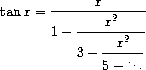where x is in radians. Define a procedure (tan-cf x k) that computes an approximation to the tangent function based on Lambert's formula. K specifies the number of terms to compute, as in exercise 1.37.

### 1.3.4  Procedures as Returned Values

The above examples demonstrate how the ability to pass procedures as arguments significantly enhances the expressive power of our programming language. We can achieve even more expressive power by creating procedures whose returned values are themselves procedures.

We can illustrate this idea by looking again at the fixed-point example described at the end of section 1.3.3. We formulated a new version of the square-root procedure as a fixed-point search, starting with the observation that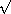x is a fixed-point of the function yx/y. Then we used average damping to make the approximations converge. Average damping is a useful general technique in itself. Namely, given a function f, we consider the function whose value at x is equal to the average of x and f(x).

We can express the idea of average damping by means of the following procedure:

(define (average-damp f)
(lambda (x) (average x (f x))))

Average-damp is a procedure that takes as its argument a procedure f and returns as its value a procedure (produced by the lambda) that, when applied to a number x, produces the average of x and (f x). For example, applying average-damp to the square procedure produces a procedure whose value at some number x is the average of x and x2. Applying this resulting procedure to 10 returns the average of 10 and 100, or 55:59

((average-damp square) 10)
55

Using average-damp, we can reformulate the square-root procedure as follows:

(define (sqrt x)
(fixed-point (average-damp (lambda (y) (/ x y)))
1.0))

Notice how this formulation makes explicit the three ideas in the method: fixed-point search, average damping, and the function yx/y. It is instructive to compare this formulation of the square-root method with the original version given in section 1.1.7. Bear in mind that these procedures express the same process, and notice how much clearer the idea becomes when we express the process in terms of these abstractions. In general, there are many ways to formulate a process as a procedure. Experienced programmers know how to choose procedural formulations that are particularly perspicuous, and where useful elements of the process are exposed as separate entities that can be reused in other applications. As a simple example of reuse, notice that the cube root of x is a fixed point of the function yx/y2, so we can immediately generalize our square-root procedure to one that extracts cube roots:60

(define (cube-root x)
(fixed-point (average-damp (lambda (y) (/ x (square y))))
1.0))

#### Newton's method

When we first introduced the square-root procedure, in section 1.1.7, we mentioned that this was a special case of Newton's method. If xg(x) is a differentiable function, then a solution of the equation g(x) = 0 is a fixed point of the function xf(x) where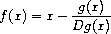and Dg(x) is the derivative of g evaluated at x. Newton's method is the use of the fixed-point method we saw above to approximate a solution of the equation by finding a fixed point of the function f.61 For many functions g and for sufficiently good initial guesses for x, Newton's method converges very rapidly to a solution of g(x) = 0.62

In order to implement Newton's method as a procedure, we must first express the idea of derivative. Note that ``derivative,'' like average damping, is something that transforms a function into another function. For instance, the derivative of the function xx3 is the function x3x2. In general, if g is a function and dx is a small number, then the derivative Dg of g is the function whose value at any number x is given (in the limit of small dx) by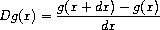Thus, we can express the idea of derivative (taking dx to be, say, 0.00001) as the procedure

(define (deriv g)
(lambda (x)
(/ (- (g (+ x dx)) (g x))
dx)))

along with the definition

(define dx 0.00001)

Like average-damp, deriv is a procedure that takes a procedure as argument and returns a procedure as value. For example, to approximate the derivative of xx3 at 5 (whose exact value is 75) we can evaluate

(define (cube x) (* x x x))
((deriv cube) 5)
75.00014999664018

With the aid of deriv, we can express Newton's method as a fixed-point process:

(define (newton-transform g)
(lambda (x)
(- x (/ (g x) ((deriv g) x)))))
(define (newtons-method g guess)
(fixed-point (newton-transform g) guess))

The newton-transform procedure expresses the formula at the beginning of this section, and newtons-method is readily defined in terms of this. It takes as arguments a procedure that computes the function for which we want to find a zero, together with an initial guess. For instance, to find the square root of x, we can use Newton's method to find a zero of the function yy2 - x starting with an initial guess of 1.63 This provides yet another form of the square-root procedure:

(define (sqrt x)
(newtons-method (lambda (y) (- (square y) x))
1.0))

#### Abstractions and first-class procedures

We've seen two ways to express the square-root computation as an instance of a more general method, once as a fixed-point search and once using Newton's method. Since Newton's method was itself expressed as a fixed-point process, we actually saw two ways to compute square roots as fixed points. Each method begins with a function and finds a fixed point of some transformation of the function. We can express this general idea itself as a procedure:

(define (fixed-point-of-transform g transform guess)
(fixed-point (transform g) guess))

This very general procedure takes as its arguments a procedure g that computes some function, a procedure that transforms g, and an initial guess. The returned result is a fixed point of the transformed function.

Using this abstraction, we can recast the first square-root computation from this section (where we look for a fixed point of the average-damped version of yx/y) as an instance of this general method:

(define (sqrt x)
(fixed-point-of-transform (lambda (y) (/ x y))
average-damp
1.0))

Similarly, we can express the second square-root computation from this section (an instance of Newton's method that finds a fixed point of the Newton transform of yy2 - x) as

(define (sqrt x)
(fixed-point-of-transform (lambda (y) (- (square y) x))
newton-transform
1.0))

We began section 1.3 with the observation that compound procedures are a crucial abstraction mechanism, because they permit us to express general methods of computing as explicit elements in our programming language. Now we've seen how higher-order procedures permit us to manipulate these general methods to create further abstractions.

As programmers, we should be alert to opportunities to identify the underlying abstractions in our programs and to build upon them and generalize them to create more powerful abstractions. This is not to say that one should always write programs in the most abstract way possible; expert programmers know how to choose the level of abstraction appropriate to their task. But it is important to be able to think in terms of these abstractions, so that we can be ready to apply them in new contexts. The significance of higher-order procedures is that they enable us to represent these abstractions explicitly as elements in our programming language, so that they can be handled just like other computational elements.

In general, programming languages impose restrictions on the ways in which computational elements can be manipulated. Elements with the fewest restrictions are said to have first-class status. Some of the ``rights and privileges'' of first-class elements are:64

• They may be named by variables.
• They may be passed as arguments to procedures.
• They may be returned as the results of procedures.
• They may be included in data structures.65

Lisp, unlike other common programming languages, awards procedures full first-class status. This poses challenges for efficient implementation, but the resulting gain in expressive power is enormous.66

Exercise 1.40.  Define a procedure cubic that can be used together with the newtons-method procedure in expressions of the form

(newtons-method (cubic a b c) 1)

to approximate zeros of the cubic x3 + ax2 + bx + c.

Exercise 1.41.  Define a procedure double that takes a procedure of one argument as argument and returns a procedure that applies the original procedure twice. For example, if inc is a procedure that adds 1 to its argument, then (double inc) should be a procedure that adds 2. What value is returned by

(((double (double double)) inc) 5)

Exercise 1.42.  Let f and g be two one-argument functions. The composition f after g is defined to be the function xf(g(x)). Define a procedure compose that implements composition. For example, if inc is a procedure that adds 1 to its argument,

((compose square inc) 6)
49

Exercise 1.43.  If f is a numerical function and n is a positive integer, then we can form the nth repeated application of f, which is defined to be the function whose value at x is f(f(...(f(x))...)). For example, if f is the function xx + 1, then the nth repeated application of f is the function xx + n. If f is the operation of squaring a number, then the nth repeated application of f is the function that raises its argument to the 2nth power. Write a procedure that takes as inputs a procedure that computes f and a positive integer n and returns the procedure that computes the nth repeated application of f. Your procedure should be able to be used as follows:

((repeated square 2) 5)
625

Hint: You may find it convenient to use compose from exercise 1.42.

Exercise 1.44.  The idea of smoothing a function is an important concept in signal processing. If f is a function and dx is some small number, then the smoothed version of f is the function whose value at a point x is the average of f(x - dx), f(x), and f(x + dx). Write a procedure smooth that takes as input a procedure that computes f and returns a procedure that computes the smoothed f. It is sometimes valuable to repeatedly smooth a function (that is, smooth the smoothed function, and so on) to obtained the n-fold smoothed function. Show how to generate the n-fold smoothed function of any given function using smooth and repeated from exercise 1.43.

Exercise 1.45.  We saw in section 1.3.3 that attempting to compute square roots by naively finding a fixed point of yx/y does not converge, and that this can be fixed by average damping. The same method works for finding cube roots as fixed points of the average-damped yx/y2. Unfortunately, the process does not work for fourth roots -- a single average damp is not enough to make a fixed-point search for yx/y3 converge. On the other hand, if we average damp twice (i.e., use the average damp of the average damp of yx/y3) the fixed-point search does converge. Do some experiments to determine how many average damps are required to compute nth roots as a fixed-point search based upon repeated average damping of yx/yn-1. Use this to implement a simple procedure for computing nth roots using fixed-point, average-damp, and the repeated procedure of exercise 1.43. Assume that any arithmetic operations you need are available as primitives.

Exercise 1.46.  Several of the numerical methods described in this chapter are instances of an extremely general computational strategy known as iterative improvement. Iterative improvement says that, to compute something, we start with an initial guess for the answer, test if the guess is good enough, and otherwise improve the guess and continue the process using the improved guess as the new guess. Write a procedure iterative-improve that takes two procedures as arguments: a method for telling whether a guess is good enough and a method for improving a guess. Iterative-improve should return as its value a procedure that takes a guess as argument and keeps improving the guess until it is good enough. Rewrite the sqrt procedure of section 1.1.7 and the fixed-point procedure of section 1.3.3 in terms of iterative-improve.

49 This series, usually written in the equivalent form (/4) = 1 - (1/3) + (1/5) - (1/7) + ···, is due to Leibniz. We'll see how to use this as the basis for some fancy numerical tricks in section 3.5.3.

50 Notice that we have used block structure (section 1.1.8) to embed the definitions of pi-next and pi-term within pi-sum, since these procedures are unlikely to be useful for any other purpose. We will see how to get rid of them altogether in section 1.3.2.

51 The intent of exercises 1.31-1.33 is to demonstrate the expressive power that is attained by using an appropriate abstraction to consolidate many seemingly disparate operations. However, though accumulation and filtering are elegant ideas, our hands are somewhat tied in using them at this point since we do not yet have data structures to provide suitable means of combination for these abstractions. We will return to these ideas in section 2.2.3 when we show how to use sequences as interfaces for combining filters and accumulators to build even more powerful abstractions. We will see there how these methods really come into their own as a powerful and elegant approach to designing programs.

52 This formula was discovered by the seventeenth-century English mathematician John Wallis.

53 It would be clearer and less intimidating to people learning Lisp if a name more obvious than lambda, such as make-procedure, were used. But the convention is firmly entrenched. The notation is adopted from thecalculus, a mathematical formalism introduced by the mathematical logician Alonzo Church (1941). Church developed thecalculus to provide a rigorous foundation for studying the notions of function and function application. Thecalculus has become a basic tool for mathematical investigations of the semantics of programming languages.

54 Understanding internal definitions well enough to be sure a program means what we intend it to mean requires a more elaborate model of the evaluation process than we have presented in this chapter. The subtleties do not arise with internal definitions of procedures, however. We will return to this issue in section 4.1.6, after we learn more about evaluation.

55 We have used 0.001 as a representative ``small'' number to indicate a tolerance for the acceptable error in a calculation. The appropriate tolerance for a real calculation depends upon the problem to be solved and the limitations of the computer and the algorithm. This is often a very subtle consideration, requiring help from a numerical analyst or some other kind of magician.

56 This can be accomplished using error, which takes as arguments a number of items that are printed as error messages.

57 Try this during a boring lecture: Set your calculator to radians mode and then repeatedly press the cos button until you obtain the fixed point.

58(pronounced ``maps to'') is the mathematician's way of writing lambda. yx/y means (lambda(y) (/ x y)), that is, the function whose value at y is x/y.

59 Observe that this is a combination whose operator is itself a combination. Exercise 1.4 already demonstrated the ability to form such combinations, but that was only a toy example. Here we begin to see the real need for such combinations -- when applying a procedure that is obtained as the value returned by a higher-order procedure.

60 See exercise 1.45 for a further generalization.

61 Elementary calculus books usually describe Newton's method in terms of the sequence of approximations xn+1 = xn - g(xn)/Dg(xn). Having language for talking about processes and using the idea of fixed points simplifies the description of the method.

62 Newton's method does not always converge to an answer, but it can be shown that in favorable cases each iteration doubles the number-of-digits accuracy of the approximation to the solution. In such cases, Newton's method will converge much more rapidly than the half-interval method.

63 For finding square roots, Newton's method converges rapidly to the correct solution from any starting point.

64 The notion of first-class status of programming-language elements is due to the British computer scientist Christopher Strachey (1916-1975).

65 We'll see examples of this after we introduce data structures in chapter 2.

66 The major implementation cost of first-class procedures is that allowing procedures to be returned as values requires reserving storage for a procedure's free variables even while the procedure is not executing. In the Scheme implementation we will study in section 4.1, these variables are stored in the procedure's environment.